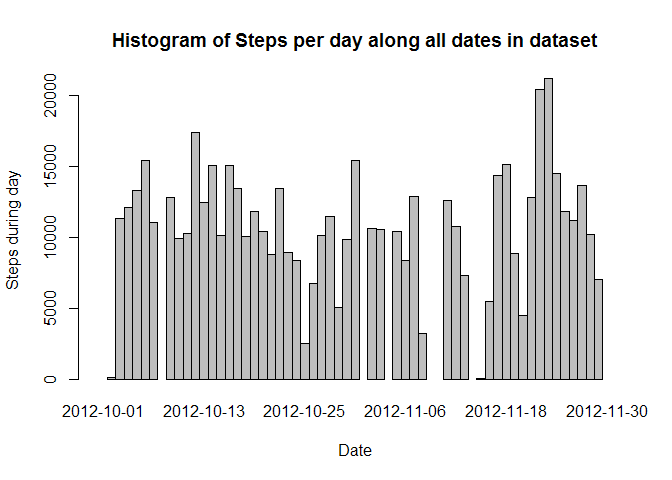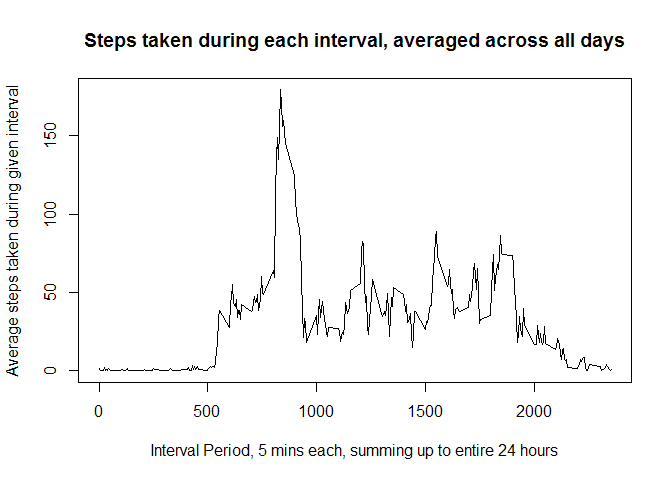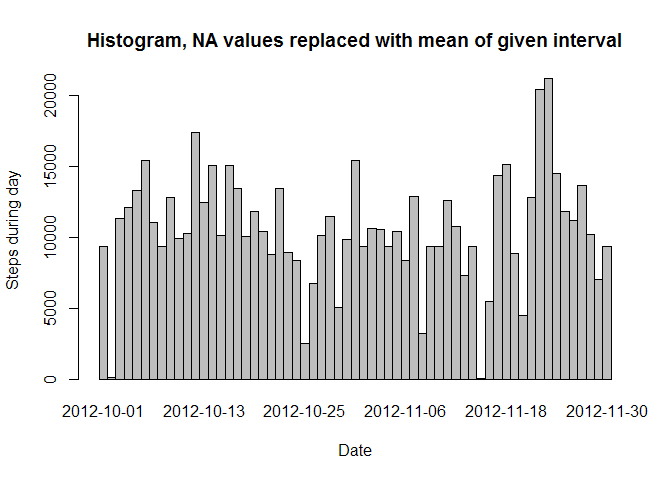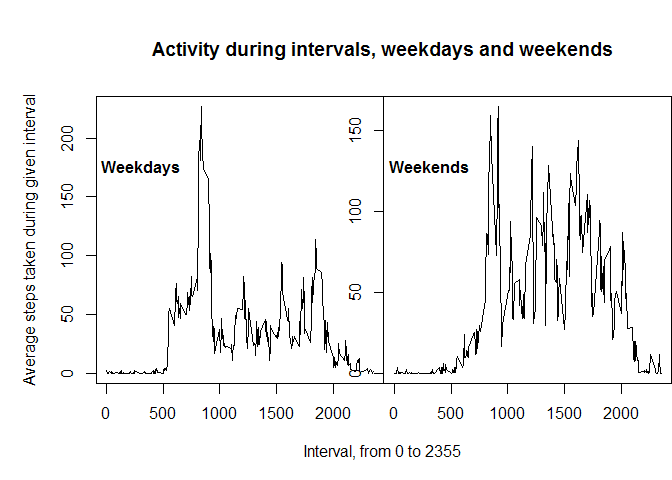# Analyzing Pedometer Data with R

``library(dplyr)``
``data <- read.csv("activity.csv")``

## What is mean total number of steps taken per day?

We first group the data by date, then collapse the steps rows into each day using the sum function as below:

``````grouped.by.day <- group_by(data, date)
steps.daily.summed <- summarise_each(grouped.by.day, funs(sum))

barplot(steps.daily.summed\$steps, names.arg = steps.daily.summed\$date,
xlab = 'Date', ylab = 'Steps during day', space = 0,
main = 'Histogram of Steps per day along all dates in dataset')``````### Finding the mean and median of steps per day

``mean(steps.daily.summed\$steps, na.rm = TRUE)``
``##  10766``
``median(steps.daily.summed\$steps, na.rm = TRUE)``
``##  10765``

We can see that the mean is 10766 and the median 10765.

## What is the average daily activity pattern?

To find this we first set all NA’s in step values to 0 in a copy of our original data, then group by intervals and find the mean on all steps in a given interval.

``````data2 <- data
data2[is.na(data2)] <- 0
grouped.by.interval <- group_by(data2, interval)
interval.alldays.mean <- summarise_each(grouped.by.interval, funs(mean))

plot(interval.alldays.mean\$interval, interval.alldays.mean\$steps, type="l",
xlab = "Interval Period, 5 mins each, summing up to entire 24 hours",
ylab = "Average steps taken during given interval",
main = "Steps taken during each interval, averaged across all days")``````We can zoom in on which precise interval is producing the highest average step count via

``interval.alldays.mean[(interval.alldays.mean\$steps == max(interval.alldays.mean\$steps)),1:2]``
``````## Source: local data frame [1 x 2]
##
##     interval steps
## 104      835 179.1``````

We see that the 8:35 interval produces the most steps during the day, on average.

## Imputing missing values

``summary(data)``
``````##      steps               date          interval
##  Min.   :  0.0   2012-10-01:  288   Min.   :   0
##  1st Qu.:  0.0   2012-10-02:  288   1st Qu.: 589
##  Median :  0.0   2012-10-03:  288   Median :1178
##  Mean   : 37.4   2012-10-04:  288   Mean   :1178
##  3rd Qu.: 12.0   2012-10-05:  288   3rd Qu.:1766
##  Max.   :806.0   2012-10-06:  288   Max.   :2355
##  NA's   :2304    (Other)   :15840``````

We can see from the summary there exists 2304 rows with NA values.

We will create a copy of our original data set, find the indices where the NA values are located, and insert the average step value for the given interval, calculated previously while NA’s were set to 0.

``````data3 <- data
na.indices <- is.na(data3)
na.indices <- which(na.indices, arr.ind =  TRUE)
for (i in na.indices[,1]){
data3[i,1] <- interval.alldays.mean[interval.alldays.mean\$interval == data3[i,3],2]
}``````

We repeat our actions to create another histogram with these NA values replaced by estimates:

``````grouped.by.day <- group_by(data3, date)
steps.daily.summed <- summarise_each(grouped.by.day, funs(sum))

barplot(steps.daily.summed\$steps, names.arg = steps.daily.summed\$date,
xlab = 'Date', ylab = 'Steps during day', space = 0,
main = 'Histogram, NA values replaced with mean of given interval')``````We then recalculate the mean and median as before:

``mean(steps.daily.summed\$steps, na.rm = TRUE)``
``##  10581``
``median(steps.daily.summed\$steps, na.rm = TRUE)``
``##  10395``

These values, 10581 for the mean, and 10395 for the median, differ by being smaller than in the original dataset with NA’s. This will be heavily influenced by how we choose to make up the values to replace NA’s, and could easily have increased the mean and median if we chose differently.

## Are there differences in activity patterns between weekdays and weekends?

We first separate each day into a Weekday or a Weekend, then collapse all the columns into the average of each interval:

``````data3[!(weekdays(as.Date(data3\$date)) %in% c('Saturday', 'Sunday')),4] <- "Weekday"
data3[(weekdays(as.Date(data3\$date)) %in% c('Saturday', 'Sunday')),4] <- "Weekend"

data.weekday <- select(filter(data3, V4 == "Weekday"), interval, steps)
data.weekend <- select(filter(data3, V4 == "Weekend"), interval, steps)
grouped.weekday.interval <- group_by(data.weekday, interval)
interval.weekday.mean <- summarise_each(grouped.weekday.interval, funs(mean))
grouped.weekend.interval <- group_by(data.weekend, interval)
interval.weekend.mean <- summarise_each(grouped.weekend.interval, funs(mean))``````

We then plot the two to see if there is a difference in activity level depending on whether it is a weekday or a weekend:

``````par(mfrow = c(1,2), mar = c(0,0,0,0), oma = c(5,5,5,0))
plot(interval.weekday.mean\$interval, interval.weekday.mean\$steps, type="l")
title(outer = TRUE, ylab = "Average steps taken during given interval",
xlab = "Interval, from 0 to 2355", main = "Activity during intervals, weekdays and weekends")
title(outer = FALSE, adj = 0.025, main= "Weekdays", cex.main = 1, line = -4)
plot(interval.weekend.mean\$interval, interval.weekend.mean\$steps, type="l")
title(outer = FALSE, adj = 0.025, main= "Weekends", cex.main = 1, line = -4)``````One can see that activity on weekdays often starts near 5-6 AM, peaks near 8:30, then is moderate for the rest of the day. On the contrary, activity during weekends starts later and is very volatile throughout the whole day.